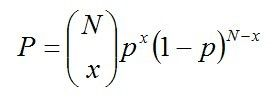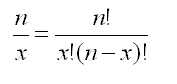# Binomial Distribution

A common probability distribution that models the probability of obtaining one of two outcomes under a given number of parameters

## What is Binomial Distribution?

Binomial distribution is a common probability distribution that models the probability of obtaining one of two outcomes under a given number of parameters. It summarizes the number of trials when each trial has the same chance of attaining one specific outcome. The value of binomial is obtained by multiplying the number of independent trials by the successes.For example, when tossing a coin, the probability of obtaining a head is 0.5. If there are 50 trials, the expected value of the number of heads is 25 (50 x 0.5). The binomial distribution is used in statistics as a building block for dichotomous variables such as the likelihood that either candidate A or B will emerge in position 1 in the midterm exams.

### Criteria of Binomial Distribution

Binomial distribution models the probability of occurrence of an event when the specific criteria are met. Binomial distribution involves the following rules that must be present in the process in order to use the binomial probability formula:

#### 1. Fixed trials

The process under investigation must have a fixed number of trials that cannot be altered in the course of the analysis. During the analysis, each trial must be performed in a uniform manner, although each trial may yield a different outcome.

In the binomial probability formula, the number of trials is represented by the letter “n.” An example of a fixed trial may be coin flips, free throws, wheel spins, etc. The number of times that each trial is conducted is known from the start. If a coin is flipped 10 times, each flip of the coin is a trial.

#### 2. Independent trials

The other condition of a binomial probability is that the trials are independent of each other. In simple terms, the outcome of one trial should not affect the outcome of the subsequent trials.

When using certain sampling methods, there is a possibility of having trials that are not completely independent of each other, and binomial distribution may only be used when the size of the population is large vis-a-vis the sample size.

An example of independent trials may be tossing a coin or rolling a dice. When tossing a coin, the first event is independent of the subsequent events.

### 3. Fixed probability of success

In a binomial distribution, the probability of getting a success must remain the same for the trials we are investigating. For example, when tossing a coin, the probability of flipping a coin is ½ or 0.5 for every trial we conduct, since there are only two possible outcomes.

In some sampling techniques like sampling without replacement, the probability of success from each trial may vary from one trial to the other. For example, assume that there are 50 boys in a population of 1,000 students. The probability of picking a boy from that population is 0.05.

In the next trial, there will be 49 boys out of 999 students. The probability of picking a boy in the next trial is 0.049. It shows that in subsequent trials, the probability from one trial to the next will vary slightly from the prior trial.

#### 4. Two mutually exclusive outcomes

In binomial probability, there are only two mutually exclusive outcomes, i.e., success or failure. While success is generally a positive term, it can be used to mean that the outcome of the trial agrees with what you have defined as a success, whether it is a positive or negative outcome.

For example, when a business receives a consignment of lamps with a lot of breakages, the business can define success for the trial to be every lamp that has broken glass. A failure can be defined as when the lamps have zero broken glasses.

In our example, the instances of broken lamps may be used to denote success as a way of showing that a high proposition of the lamps in the consignment is broken. and that there is a low probability of getting a lamp with zero breakages.

### Example of Binomial Distribution

Suppose, according to the latest police reports, 80% of all petty crimes are unresolved, and in your town, at least three of such petty crimes are committed. The three crimes are all independent of each other. From the given data, what is the probability that one of the three crimes will be resolved?

#### Solution

The first step in finding the binomial probability is to verify that the situation satisfies the four rules of binomial distribution:

• Number of fixed trials (n): 3 (Number of petty crimes)
• Number of mutually exclusive outcomes: 2 (solved and unsolved)
• The probability of success (p): 0.2 (20% of cases are solved)
• Independent trials: Yes

Next:

We find the probability that one of the crimes will be solved in the three independent trials. It is shown as follows:

Trial 1 = Solved 1st, unsolved 2nd, and unsolved 3rd

= 0.2 x 0. 8 x 0.8

= 0.128

Trial 2 = Unsolved 1st, solved 2nd, and unsolved 3rd

= 0.8 x 0.2 x 0.8

= 0.128

Trial 3 = Unsolved 1st, unsolved 2nd, and solved 3rd

= 0.8 x 0.8 x 0.2

= 0.128

Total (for the three trials):

= 0.128 + 0.128 + 0.128

= 0.384

Alternatively, we can apply the information in the binomial probability formula, as follows:Where:In the equation, x = 1 and n = 3. The equation gives a probability of 0.384.